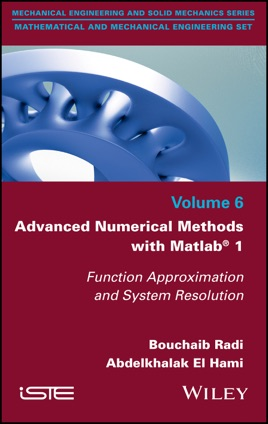• USD 114.99

## Descripción de editorial

Most physical problems can be written in the form of mathematical equations (differential, integral, etc.). Mathematicians have always sought to find analytical solutions to the equations encountered in the different sciences of the engineer (mechanics, physics, biology, etc.). These equations are sometimes complicated and much effort is required to simplify them. In the middle of the 20th century, the arrival of the first computers gave birth to new methods of resolution that will be described by numerical methods. They allow solving numerically as precisely as possible the equations encountered (resulting from the modeling of course) and to approach the solution of the problems posed. The approximate solution is usually computed on a computer by means of a suitable algorithm.

The objective of this book is to introduce and study the basic numerical methods and those advanced to be able to do scientific computation. The latter refers to the implementation of approaches adapted to the treatment of a scientific problem arising from physics (meteorology, pollution, etc.) or engineering (structural mechanics, fluid mechanics, signal processing, etc.) .